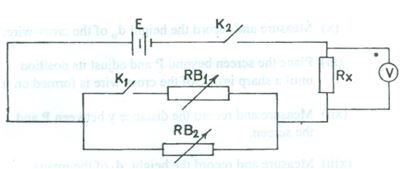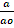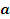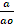Physics Paper 3, May/June 2014
 Questions: 1 2 3 Main
General Comments
Weakness/Remedies
Strength

Question 2

(a)You are provided with an illuminated object, converging lens, screen, metre rule and other necessary materials.

1. Measure and record the size ao of the illuminated object.
2. Place the object O and the screen S on opposite sides of the converging lens L.
3. Set the distance between the object and the lens U = 30 cm.
4. Adjust the screen until a sharp image of the illuminated object is obtained on the screen.
5. Measure and record the size a of the image.
6. Evaluate m =and m-1
7. Repeat the procedure for four other values of U = 35 cm, 40 cm, 45 cm and 50 cm respectively.
8. Tabulate your readings.
9. Plot a graph with m-1 on the vertical axis and U on the horizontal axis.
10. Determine the slope, s, of the graph and intercept, e, on the vertical axis.
11. Determine the value of U for which m-1 = 0;
12. State two precautions taken to obtain accurate results.

(a)       (i)     Using your graph, determine the value of m for which U = 37 cm.

(ii)       Sketch a diagram to illustrate how a converging lens may be used to produce a real diminished image of an object.

_____________________________________________________________________________________________________
Observation

Part (a)  This was a fairly popular question among the candidates.  Candidates were instructed to measure and record ao.   However, many responding candidates did not record this value but still calculated the quantity m =.   They scored zero for this column.  Intercept was to be determined on the vertical axis, however, majority of the candidates got it on the horizontal axis.  Hence, they could not determine the value of M for which U = 37 cm.  The few that got the intercept correctly failed to realize that the value of  m can be obtained from the value of m-1.   Few candidates even found it difficult to plot the graph due to the small values of m-1.

Part (b).  Many could not draw the ray diagram.

The expected answers are that candidates should:

•  Measure and record value of ao to at least 1 d.p in cm
• Measure and record five  values of U to at least 1 d.p in cm

(iii)       Measure and record five values ofto at least1 d.p in cm and in trend
(iv)       Correctly evaluate five values of m =to at least 2 d.p
(v)        Correctly evaluate five values of m-1 to at least 3 s.f.

• Show composite table containing U, a, m and m-1
• Plot graph using reasonable scales
• Draw line of best fit
• Determine the slope of the graph and the intercept
• Determine the value of U for which m-1 = 0
• State any two of the following precautions

e.g  -   Avoided parallax error on metre rule/rule.
-   Ensured that the arrangement of apparatus are co-axial
-   Repeated readings (shown on table)

In part (b), the expected answers are:

(i) m-1  correctly traced from u – 37cm

m correctly evaluated

(ii)To score

1. Object must be beyond 2f.
2. Correct image must be between f and 2f on the other side.
3. Any two correct rays from the object intersecting to form the image.

 Powered by Sidmach Technologies(Nigeria) Limited . Copyright © 2015 The West African Examinations Council. All rights reserved.